Blog / ISHIDO-SHIKI / Math problems [for those who want to be strong in numbers]
Math problems [for those who want to be strong in numbers]

10 Jan,2020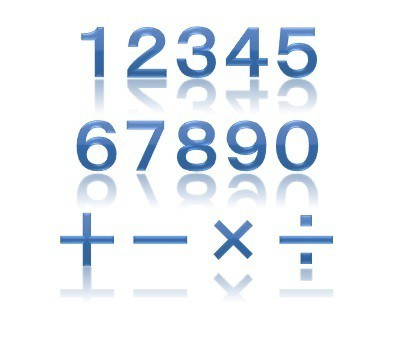For those who want to be strong in math problems.
Those who do not understand the basics of mathematics properly and want to learn again.
Also, are you looking for ways and places to learn from the basics?

I'm the author of this article and I'm a qualified abacus teacher, answering questions from around the world online and various questions from online abacus.com students.

1.Learn the basics of math question
2.Solve math practice using arithmetic calculations

1.Learn the basics of math question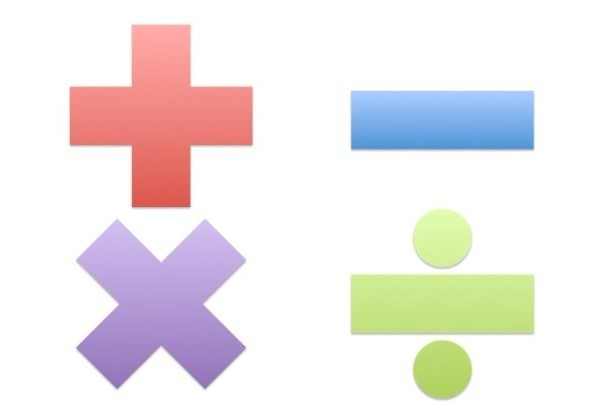1-1. What are the four arithmetic calculations?
1-2.The calculation method of the four arithmetic calculations

1.Learn the basics of math question
1-1.What are the four arithmetic calculations?
You will be able to understand what arithmetic is. If you are not sure, please take a look.
The four arithmetics are important because they form the basis of many mathematical practice.
Introducing four [+-× ÷] that are essential for solving math problems.

2-1.Calculation method of the four arithmetic calculations
You will understand the rules of the four arithmetic calculations.
First of all, please use when you do not know the small child from the rules of numbers.
I will explain while actually looking at the calculation problem.

Solve math practice using arithmetic calculations2-1.＋ Math problems
2-2.－ Math problems
2-3.×  Math problems
2-4.÷  Math problems
2-5. Combination math problems

2.Solve math practice using arithmetic calculations

2-1. ＋Math practiceYou will be able to understand the addition.
I try to do simple additions and understand the concept of numbers while looking at a table of number friends. I write the images as much as possible so that I can understand the numbers of small children, such as which of the numbers is larger or smaller.
First, look at the images and remember your number friends.

2-2.－Math practiceYou will be able to understand the subtraction.
I do simple subtraction and understand the concept of numbers while looking at a table of number friends. I write the images as much as possible so that I can understand the numbers of small children, such as which of the numbers is larger or smaller.
First, look at the images and remember your number friends.

2-3. × Math practice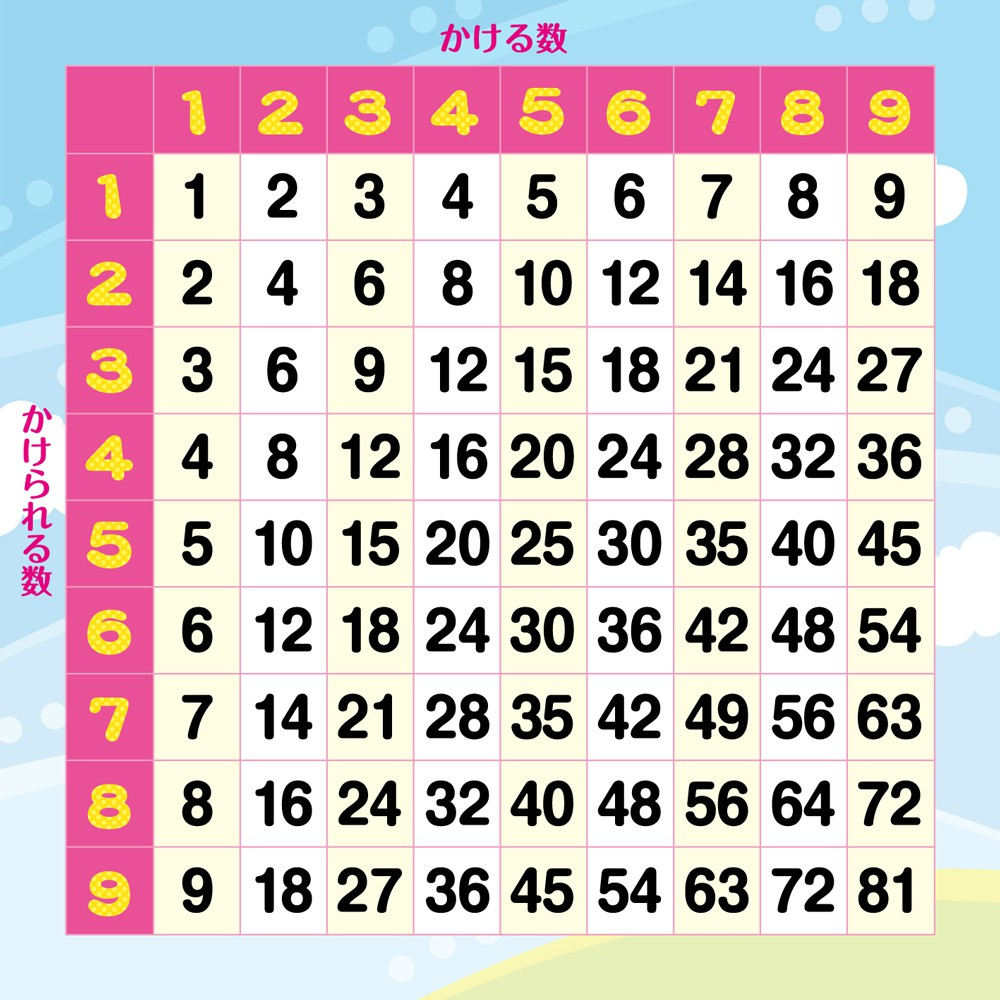You will be able to understand the multiplication.
Practice to multiply 99.
First, look at the images and remember your number friends.

2-4.÷ Math practiceYou will understand division.
If the multiplication 99 is firm, any four arithmetic calculations can be done.
Look at the image and think about it.

2-5.Combination math practice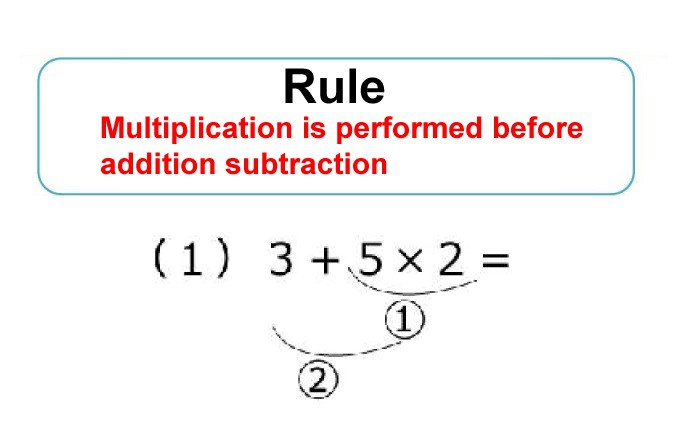You will be able to understand the problem of various arithmetic calculations.
"There are rules in the four arithmetic calculations.
Let's look at the order in which the calculations should be performed while actually looking at the calculation problem in which +-× ÷ is mixed.
If you make a mistake in the calculation order, you will get a different answer."
Let's see where to calculate from the image.

"Do you now understand the four arithmetic calculations?
It takes practice to understand the answer instantly.
If you want to be better, go to ISHIDO-SHIKI
It is an online course of ISHIDO-SHIKI which has produced many winners and runners up in the mental arithmetic world cup."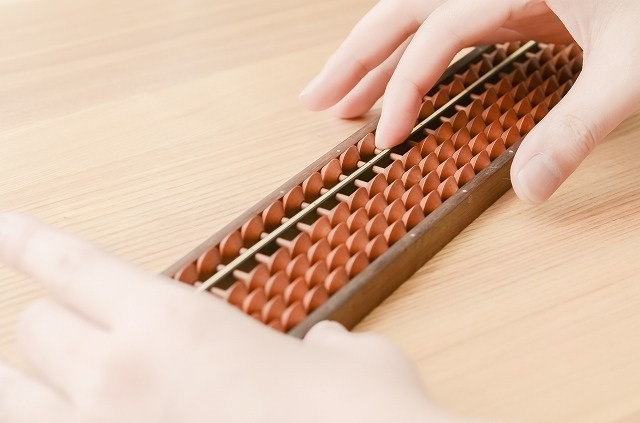There are various types of abacus, but from the perspective of abacus teachers and professionalsLet's try Abacus online competition
This article is for those who want to abacus online competition. If you are confident in the abacus and want to compete with the world, please take a look. This site allows you to compete online with people from around the world by mental arithmetic using the power of the abacus.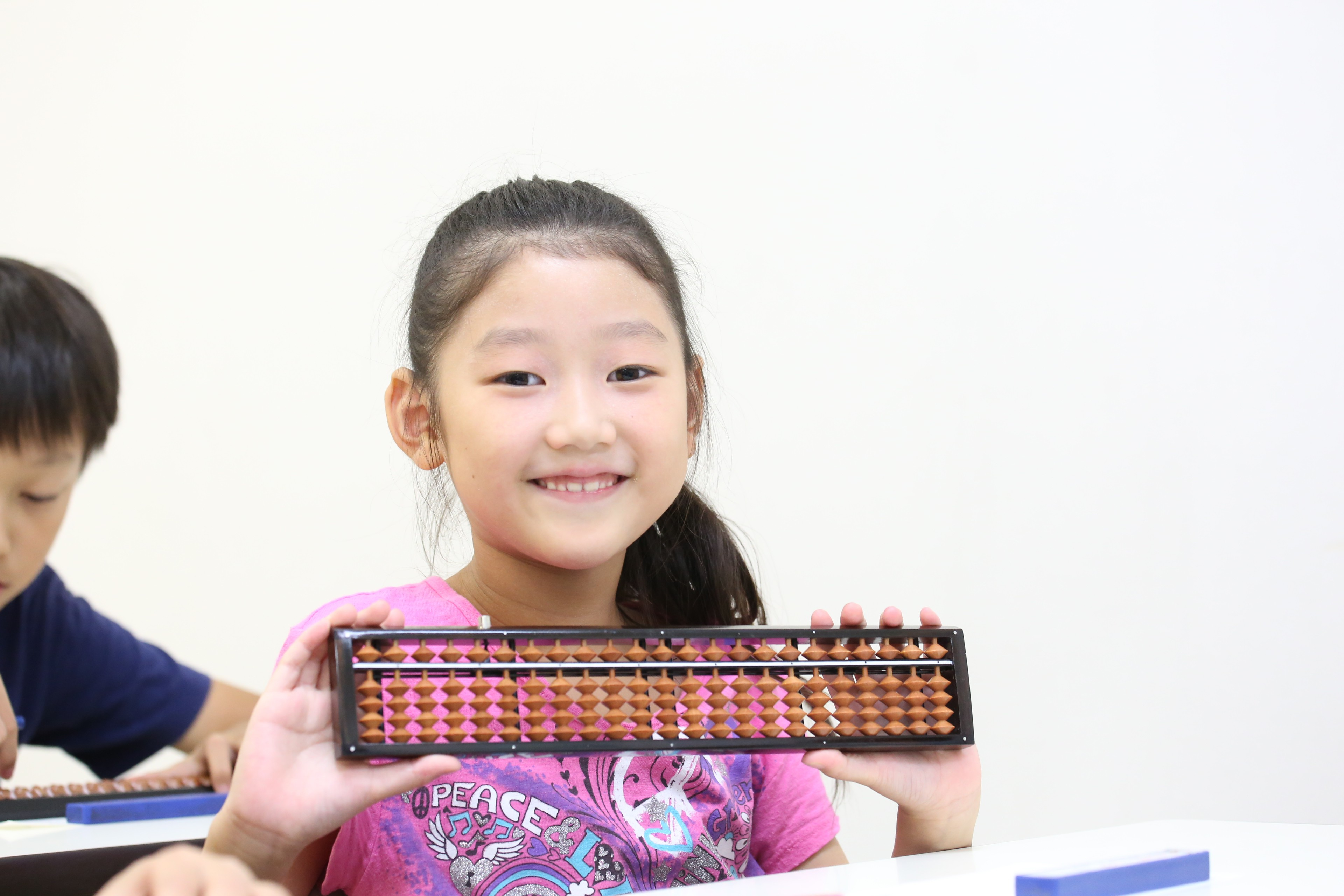Same categoryLet's learning ISHIDO-SHIKI